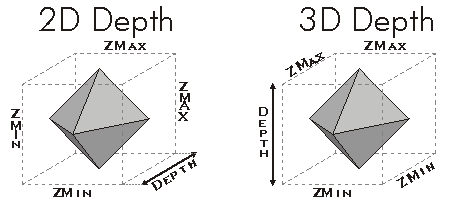ArcObjects Library Reference (Geometry)

# IEnvelope.ZMax Property

The maximum Z value in the area of the envelope.

```[Visual Basic .NET]
Public Property ZMax As Double```
```[C#]
public double ZMax {get; set;}```
```[C++]
HRESULT get_ZMax(
double* ZMax
);```
```[C++]
HRESULT put_ZMax(
double ZMax
);```
```[C++]
Parameters
ZMax [out, retval]
ZMax is a parameter of type double
ZMax
ZMax is a parameter of type double
```

#### Product Availability

Available with ArcGIS Engine, ArcGIS Desktop, and ArcGIS Server.

#### Description

ZMax defines the upper elevation boundary of the envelope.

#### Remarks[C#]

The following C# code shows how to read and write the ZMax value:

IEnvelope env = new EnvelopeClass() as IEnvelope;

env.ZMax = 2;

double zMax;

zMax = env.ZMax;

#### See Also

IEnvelope Interface